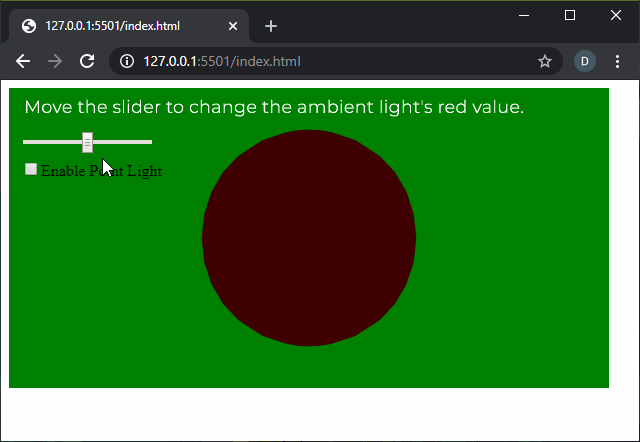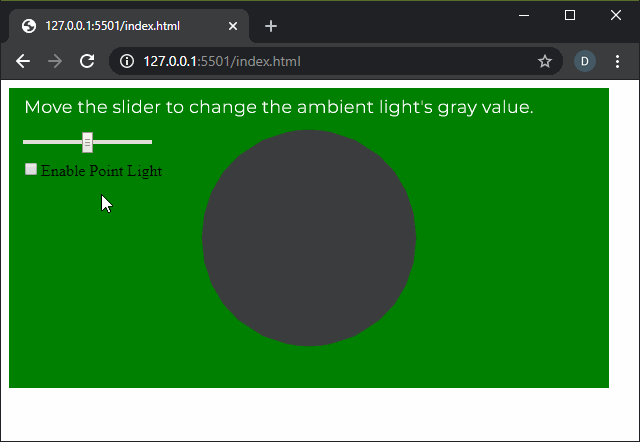# p5.js | ambientLight() Function

• Last Updated : 27 Apr, 2020

The ambientLight() function in p5.js is used to create an ambient light with the specified color. Ambient light does not have any particular source. It comes from everywhere in the canvas and illuminates objects evenly.

Syntax:

`ambientLight( v1, v2, v3, [alpha] )`

OR

`ambientLight( value )`

OR

`ambientLight( gray, [alpha] )`

OR

`ambientLight( values )`

OR

`ambientLight( color )`

Parameters: This function accept eight parameters as mentioned above and described below:

• v1: It is a number which determines the red or hue value relative to the current color range.
• v2: It is a number which determines the green or saturation value relative to the current color range.
• v3: It is a number which determines the blue or brightness value relative to the current color range.
• alpha: It is a number which determines the alpha value of the color.
• value: It is a string which defines the ambient light color.
• gray: It is a number which defines the gray value of the ambient light.
• values: It is an array of numbers which define the red, green, blue and alpha components of the ambient light color.
• color: It is a p5.Color which defines the color of the ambient light.

Below examples illustrates the ambientLight() function in p5.js:

Example 1:

 `let newFont;``let pointLightEnable = ``false``;`` ` `function` `preload() {``  ``newFont = loadFont(``'fonts/Montserrat.otf'``);``}`` ` `function` `setup() {``  ``createCanvas(600, 300, WEBGL);``  ``textFont(newFont, 18);`` ` `  ``graySlider = createSlider(0, 128, 64, 1);``  ``graySlider.position(20, 50);`` ` `  ``pointLightCheck = createCheckbox(``       ``"Enable Point Light"``, ``false``);`` ` `  ``pointLightCheck.position(20, 80);`` ` `  ``// Toggle point light``  ``pointLightCheck.changed(() => {``    ``pointLightEnable = !pointLightEnable;``  ``});``}`` ` `function` `draw() {``  ``background(``"green"``);``  ``text(``"Move the slider to change the ambient"``        ``+ ``" light's red value."``, -285, -125);``  ``noStroke();``  ``shininess(15);``  ``if` `(pointLightEnable) {``    ``pointLight(0, 0, 255, -width / 2,``                    ``-height / 2, 250);``  ``}`` ` `  ``grayValue = graySlider.value();``  ``ambientLight(grayValue, 0, 0);``  ``specularMaterial(250);``  ``sphere(100);``}`

Output:Example 2:

 `let newFont;``let pointLightEnable = ``false``;`` ` `function` `preload() {``  ``newFont = loadFont(``'fonts/Montserrat.otf'``);``}`` ` `function` `setup() {``  ``createCanvas(600, 300, WEBGL);``  ``textFont(newFont, 18);`` ` `  ``graySlider = createSlider(0, 128, 64, 1);``  ``graySlider.position(20, 50);`` ` `  ``pointLightCheck = createCheckbox(``        ``"Enable Point Light"``, ``false``);`` ` `  ``pointLightCheck.position(20, 80);`` ` `  ``// Toggle point light``  ``pointLightCheck.changed(() => {``    ``pointLightEnable = !pointLightEnable;``  ``});``}`` ` `function` `draw() {``  ``background(``"green"``);``  ``text(``"Move the slider to change the ambient"``      ``+ ``" light's gray value."``, -285, -125);``  ``noStroke();``  ``shininess(15);``  ``if` `(pointLightEnable) {``    ``pointLight(255, 0, 0, -width / 2,``                    ``-height / 2, 250);``  ``}`` ` `  ``grayValue = graySlider.value();``  ``ambientLight(grayValue);``  ``specularMaterial(250);``  ``sphere(100);``}`

Output:Online editor: https://editor.p5js.org/

My Personal Notes arrow_drop_up